Open in App
Not now

# Image classification using CIFAR-10 and CIFAR-100 Dataset in TensorFlow

• Last Updated : 21 Nov, 2022

CIFAR10 and CIFAR100 are some of the famous benchmark datasets which are used to train CNN for the computer vision task.

In this article we are supposed to perform image classification on both of these datasets CIFAR10 as well as CIFAR100 so, we will be using Transfer learning here.

But how? First, we will build a custom CNN model and train it on our CIFAR100 data. And then we will leverage the trained weights of the Convolutional layers to build a classification model for the CIFAR10 dataset.

### Importing Libraries

We will be requiring the following libraries :

## Python3

 `import` `tensorflow as tf ` `from` `tensorflow ``import` `keras ` `from` `keras ``import` `layers ` ` `  `import` `numpy as np ` `import` `matplotlib.pyplot as plt ` ` `  `import` `warnings ` `warnings.filterwarnings(``'ignore'``)`

### Importing Dataset

Now let’s load the CIFAR100 dataset first by using the TensorFlow API. The data fetched is already divided into training and validation datasets.

## Python3

 `# Load in the data ` `cifar100 ``=` `tf.keras.datasets.cifar100 ` ` `  `# Distribute it to train and test set ` `(x_train, y_train), (x_val, y_val) ``=` `cifar100.load_data() ` `print``(x_train.shape, y_train.shape, x_val.shape, y_val.shape) `

Output:

```Downloading data from https://www.cs.toronto.edu/~kriz/cifar-100-python.tar.gz
169001437/169001437 [==============================] - 13s 0us/step
(50000, 32, 32, 3) (50000, 1) (10000, 32, 32, 3) (10000, 1)```

This means that we have 50,000 RGB format images of sizes (32, and 32) in the training data. And around 10,000 images in the validation dataset.

### Data Visualization

Here in this step we will visualize the dataset, it will further help us to make a better model.

## Python3

 `def` `show_samples(data, labels): ` `    ``plt.subplots(figsize``=``(``10``, ``10``)) ` `    ``for` `i ``in` `range``(``12``): ` `        ``plt.subplot(``3``, ``4``, i``+``1``) ` `        ``k ``=` `np.random.randint(``0``, data.shape[``0``]) ` `        ``plt.title(labels[k]) ` `        ``plt.imshow(data[k]) ` `    ``plt.tight_layout() ` `    ``plt.show() ` ` `  ` `  `show_samples(x_train, y_train) `

Output: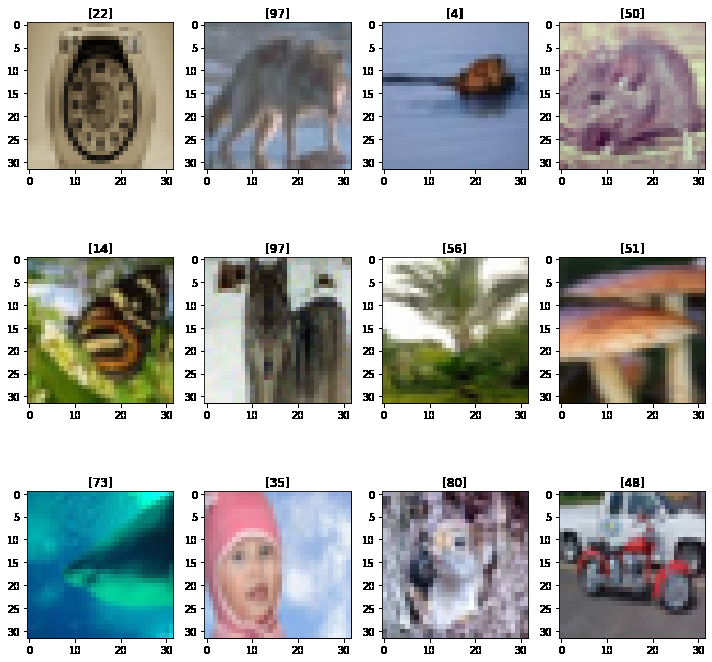Due to the very small size of the images, their content is not that clear. To know which number represent which class one can refer to various sources available online.

### Data Splitting

We need to split the data into training and validation.

## Python3

 `y_train ``=` `tf.one_hot(y_train, ` `                     ``depth``=``y_train.``max``() ``+` `1``, ` `                     ``dtype``=``tf.float64) ` `y_val ``=` `tf.one_hot(y_val, ` `                   ``depth``=``y_val.``max``() ``+` `1``, ` `                   ``dtype``=``tf.float64) ` ` `  `y_train ``=` `tf.squeeze(y_train) ` `y_val ``=` `tf.squeeze(y_val) `

### Model Architecture

Now let’s define the architecture of the model we will use as our CNN to classify between these 100 classes.

## Python3

 `model ``=` `tf.keras.models.Sequential([ ` `    ``layers.Conv2D(``16``, (``3``, ``3``), activation``=``'relu'``, ` `                  ``input_shape``=``(``32``, ``32``, ``3``), padding``=``'same'``), ` `    ``layers.Conv2D(``32``, (``3``, ``3``), ` `                  ``activation``=``'relu'``, ` `                  ``padding``=``'same'``), ` `    ``layers.Conv2D(``64``, (``3``, ``3``), ` `                  ``activation``=``'relu'``, ` `                  ``padding``=``'same'``), ` `    ``layers.MaxPooling2D(``2``, ``2``), ` `    ``layers.Conv2D(``128``, (``3``, ``3``), ` `                  ``activation``=``'relu'``, ` `                  ``padding``=``'same'``), ` ` `  ` `  `    ``layers.Flatten(), ` `    ``layers.Dense(``256``, activation``=``'relu'``), ` `    ``layers.BatchNormalization(), ` `    ``layers.Dense(``256``, activation``=``'relu'``), ` `    ``layers.Dropout(``0.3``), ` `    ``layers.BatchNormalization(), ` `    ``layers.Dense(``100``, activation``=``'softmax'``) ` `]) ` ` `  `model.``compile``( ` `    ``loss``=``tf.keras.losses.CategoricalCrossentropy(from_logits``=``True``), ` `    ``optimizer``=``'adam'``, ` `    ``metrics``=``[``'AUC'``, ``'accuracy'``] ` `) `

What are the changes made to the images while moving deeper into a network that can be visualized by using the model summary? It also shows the number of parameters that will be trained in this model.

## Python3

 `model.summary()`

Output: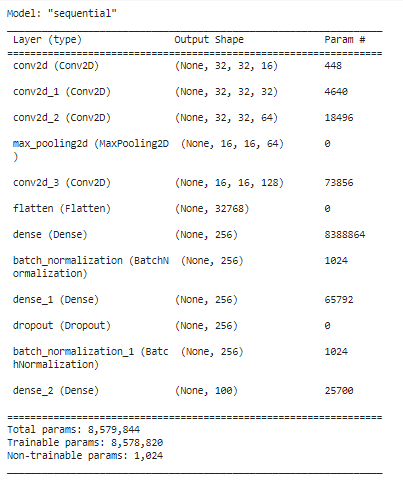### Model fitting

Model fitting can be done using the code below.

## Python3

 `hist ``=` `model.fit(x_train, y_train, ` `                 ``epochs``=``5``, ` `                 ``batch_size``=``64``, ` `                 ``verbose``=``1``, ` `                 ``validation_data``=``(x_val, y_val)) `

Output: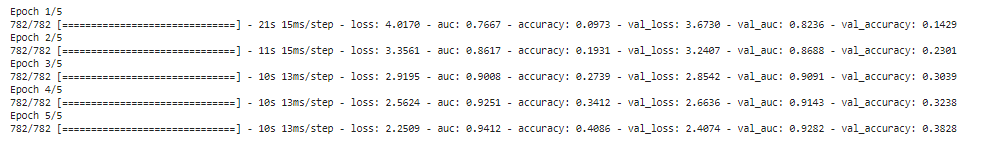### Using model to train for CIFAR10

Now we will use the convolution layers of this model to build our CIFAR10 classifier.

## Python3

 `temp ``=` `model.get_layer(``'conv2d_3'``) ` `last_output ``=` `temp.output ` `last_output.shape `

Output:

`TensorShape([None, 16, 16, 128])`

Now implementing a functional model which will use the previous model’s output and learn on top of it.

## Python3

 `x ``=` `layers.Flatten()(last_output) ` ` `  `x ``=` `layers.Dense(``256``, activation``=``'relu'``)(x) ` `x ``=` `layers.BatchNormalization()(x) ` ` `  `x ``=` `layers.Dense(``256``, activation``=``'relu'``)(x) ` `x ``=` `layers.Dropout(``0.3``)(x) ` `x ``=` `layers.BatchNormalization()(x) ` ` `  `output ``=` `layers.Dense(``10``, activation``=``'softmax'``)(x) ` ` `  `model_new ``=` `keras.Model(model.``input``, output) `

Let’s check the summary of this new model.

## Python3

 `model_new.summary() `

Output: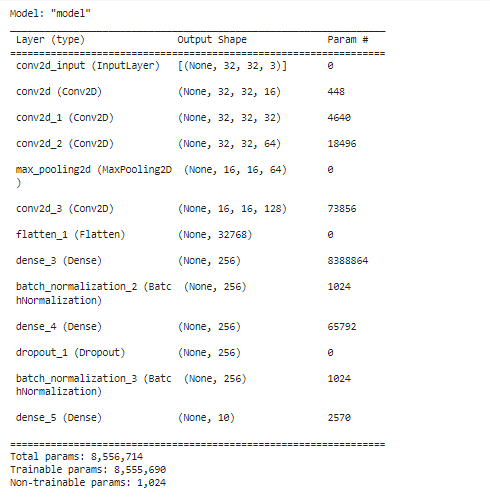Now, let’s compile the model with the same loss, optimizer, and metrics used in the CIFAR100 classifier.

## Python3

 `model_new.``compile``( ` `    ``loss``=``'categorical_crossentropy'``, ` `    ``optimizer``=``'adam'``, ` `    ``metrics``=``[``'AUC'``, ``'accuracy'``] ` `) `

So, we have prepared a new model which is now ready to be trained on the CIFAR10 dataset. Let’s load this data as well using the TensorFlow API.

## Python3

 `# Load in the data ` `cifar10 ``=` `tf.keras.datasets.cifar10 ` ` `  `# Distribute it to train and test set ` `(x_train, y_train), (x_val, y_val) ``=` `cifar10.load_data() ` `print``(x_train.shape, y_train.shape, x_val.shape, y_val.shape) `

Output:

```Downloading data from https://www.cs.toronto.edu/~kriz/cifar-10-python.tar.gz
170498071/170498071 [==============================] - 14s 0us/step
(50000, 32, 32, 3) (50000, 1) (10000, 32, 32, 3) (10000, 1)```

## Python3

 `y_train ``=` `tf.one_hot(y_train, depth``=``10``, ` `                     ``dtype``=``tf.float64) ` `y_val ``=` `tf.one_hot(y_val, depth``=``10``, ` `                   ``dtype``=``tf.float64) ` ` `  `y_train ``=` `tf.squeeze(y_train) ` `y_val ``=` `tf.squeeze(y_val) `

Now we will train our model.

## Python3

 `history ``=` `model_new.fit(x_train, y_train, ` `                        ``batch_size``=``64``, ` `                        ``epochs``=``5``, ` `                        ``verbose``=``1``, ` `                        ``validation_data``=``(x_val, y_val)) `

Output: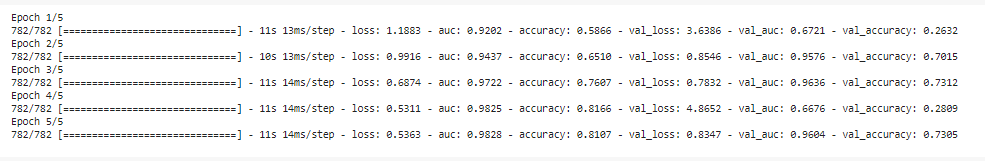### Conclusion

By training the model for more epochs the best results can be obtained.

My Personal Notes arrow_drop_up
Related Articles